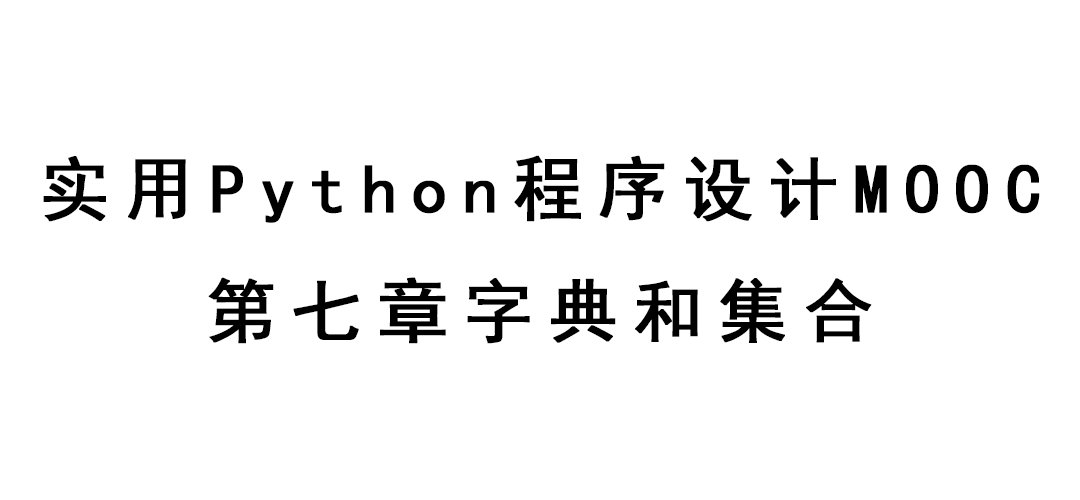[TOC]

# 字典的基本概念

## 字典

• 字典的每个元素是由”键:值”两部分组成，可以根据”键”进行快速查找
• 格式:d = {key1 : value1, key2 : value2}
• 字典元素的值是可赋值的，因此也是指针
• 所有元素的键都不相同
• 键必须是不可变的数据类型，比如字符串、整数、小数、元组。列表、集合、字典等可变的数据类型，不可作为字典元素的键。

# 字典的键不可重复

• 字典的键不可重复，指的是字典的键的内容不能一样

## 字典相关函数

clear() 清空字典
keys() 取字典的键的序列
items() 取字典的元素的序列，可用于遍历字典
values() 取字典的值序列
pop(x) 删除键为x的元素，如果不存在，产生异常

copy() 浅拷贝

• items

## 词频统计

• 输入
若干行，每行一个单词。

• 输出
按单词出现次数从高到低打出所有单词。次数相同的，按照字典序从小到大排

• 输入样例

• 输出样例

• 代码

# 集合

## 集合(set)的概念和特点

• 元素类型可以不同。
• 不会有重复元素。
• 可以增删元素。
• 整数、小数、复数、字符串、元组都可以作为集合的元素。但是列表、字典和集合等可变的数据类型不可作为集合的元素。
• 集合的作用是快速判断某个东西是否在一堆东西里面(用in)。

## 集合常用函数

add(x) 添加元素x。如果x已经存在，则不添加
clear() 清空集合
copy() 返回自身的浅拷贝
remove(x) 删除元素x。如果不存在元素x，则引发异常
update(x) 将序列x中的元素加入到集合

## 集合运算

a, b是集合

x in a x是否在集合a中
a | b 求a和b的并
a & b 求a和b的交
a - b 求a和b的差，即在a中而不在b中的元素
a ^ b 求a和b的对称差，等价于(a|b) - (a&b)

a == b a是否元素和b一样
a != b a是否元素和b不一样

a <= b a是否是b的子集(a有的元素，b都有)
a < ba是否是b的真子集(a有的元素，b都有，且b还包含a中没有的元素)
a >= bb是否是a的子集
a > b b是否是a的真子集

• 输入样例
take
zoo
take

• 输出样例
3

• 提交代码

# 程序或算法的时间复杂度

• 一个程序或算法的时间效率，也称”时间复杂度”，有时简称”复杂度”

• 复杂度常用大的字母O和小写字母n来表示，比如$O(n)$, $O(n^2)$等。n代表问题的规模，$O(X)$就表示解决问题的时间和X成正比关系。

• 时间复杂度是用算法运行过程中，某种时间固定的操作需要被执行的次数和n的关系来度量的。在无序数列中查找某个数，复杂度是$O(n)$。

• 计算复杂度的时候，只统计执行次数最多的(n足够大时)那种固定操作的次数比如某个算法需要执行加法$n^2$次，除法10000n次， 那么就记其复杂度是$O(n^2)$的。

• 如果复杂度是多个n的函数之和，则只关心随n的增长增长得最快的那个函数

• 常数复杂度: $O(1)$ 时间(操作次数)和问题的规模无关
• 对数复杂度: $O(log(n))$
• 线性复杂度: $O(n)$
• 多项式复杂度: $O(n^k)$
• 指数复杂度: $O(a^n)$
• 阶乘复杂度: $O(n!)$

• 在无序数列中查找某个数(顺序查找) $O(n)$

• 插入排序、选择排序等笨排序方法 $O(n^2)$
• 快速排序 $O(n * log(n))$
• 二分查找 $O(log(n))$

## in用于列表和用于字典、集合的区别

a in b

## 一些操作的时间复杂度总结

$O(1)$：集合、字典增删元素，查找元素，以关键字作为下标访问字典元素的值，列表添加元素到末尾(append) ，列表、字符串、元组根据下标访问元素
$O(n)$：列表、元组查找元素(in, index)， 列表插入元素(insert)、删除元素(remove)计算出现次数(count)
$O(n log(n))$：python 自带排序sort, sorted
$O(log(n))$：在排好序的列表或元组上进行二分查找(初始的查找区间是整个元组或列表，每次和查找区间中点比较大小，并缩小查找区间到原来的一半。类似于查英语词典)有序就会找得快!